# What is Half Bridge Inverter : Circuit Diagram & Its Working

The inverter is a power electronic converter that converts direct power to alternating power. By using this inverter device, we can convert fixed dc into variable ac power which as a variable frequency and voltage. Secondly from this inverter, we can vary the frequency i.e we will be able to generate the 40HZ, 50HZ, 60HZ frequencies as of our requirement. If the dc input is a voltage source then the inverter is known as VSI (Voltage Source Inverter). The inverters need four switching devices whereas half-bridge inverter needs two switching devices. The bridge inverters are of two types they are half-bridge inverter and full-bridge inverter. This article discusses the half-bridge inverter.

## What is Half-Bridge Inverter?

The inverter is a device that converts a dc voltage into ac voltage and it consists of four switches whereas half-bridge inverter requires two diodes and two switches which are connected in anti-parallel. The two switches are complementary switches which means when the first switch is ON the second switch will be OFF Similarly, when the second switch is ON the first switch will be OFF.

### Single Phase Half Bridge Inverter with Resistive Load

The circuit diagram of a single-phase half-bridge inverter with resistive load is shown in the below figure.

Where RL is the resistive load, Vs/2 is the voltage source, S1 and S2 are the two switches, i0 is the current. Where each switch is connected to diodes D1 and D2 parallelly. In the above figure, the switches S1 and S2 are the self-commutating switches. The switch S1 will conduct when the voltage is positive and current is negative, switch S2 will conduct when the voltage is negative, and the current is negative. The diode D1 will conduct when the voltage is positive and current is negative, diode D2 will conduct when the voltage is negative, and the current is positive.

Case 1 (when switch S1 is ON and S2 is OFF): When switch S1 is ON from a time period of 0 to T/2, the diode D1 and D2 are in reverse bias condition and S2 switch is OFF.

Applying KVL (Kirchhoff’s Voltage Law)

Vs/2-V0=0

Where output voltage V0= Vs/2

Where output current i0 = V0/R= Vs/2R

In case of supply current or switch current, the current iS1 = i0 = Vs/2R, iS2 = 0 and the diode current iD1 = iD2 = 0.

Case 2 (when switch S is ON and S1 is OFF): When switch S2 is ON from a time period of T/2 to T, the diode D1 and D2 are in reverse bias condition and S1 switch is OFF.

Applying KVL (Kirchhoff’s Voltage Law)

Vs/2+V0=0

Where output voltage V0= -Vs/2

Where output current i0 = V0/R= -Vs/2R

In case of supply current or switch current, the current iS1 = 0, iS2 = i0 = -Vs/2R and the diode current iD1 = iD2 = 0.

The single-phase half-bridge inverter output voltage waveform is shown in the below figure.

The average value of output voltage is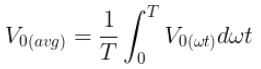So output voltage waveform from converting time ‘T’ to ‘‘ωt” axis is shown in the below figure

When is multiply by zero, it will be zero; When is multiply by T/2, it will be T/2=π; When is multiply by T, it will be T=2π; When is multiply by 3T/2, it will be T/2=3π and so on. In this way, we can convert this time axis into the ‘ωt’ axis.

The average value of output voltage and output current is

V0 (avg) = 0

I0 (avg) = 0

The RMS value of output voltage and output current is

V0 (RMS)= VS/2

I0 (RMS) = V0 (RMS) /R =VS/2R

The output voltage we are getting in an inverter is not pure sinewave i.e a square wave. The output voltage with the fundamental component is shown in the below figure.

Using Fourier series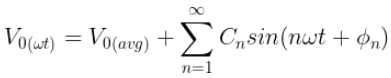Where Cn, an and bn are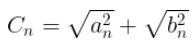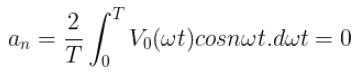bn=VS/nᴨ(1-cosnᴨ)

The bn =0 when substituting even numbers (n=2,4,6…..) and bn =2Vs/nπ when substituting odd numbers (n=1,3,5……). Substitute bn =2Vs/nπ and an=0 in Cn will get Cn=2Vs/nπ.

ϕn=tan-1(an/bn)=0

V01(ωt)=2 VS/ᴨ * (Sin ωt)

Substitute V0 (avg) = 0  in will get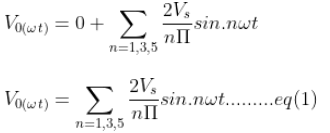The equation (1) can also be written as

V0(ωt)=2VS/ᴨ * (Sin ωt) + 2VS/3ᴨ * (Sin3ωt) +  2VS/5ᴨ * (Sin5ωt) +……..+∞

V0(ωt)= V01(ωt)+ V03(ωt)+ V05(ωt)

The above expression is the output voltage which consists of fundamental voltage and odd harmonics. There are two methods to remove these harmonic components they are: to use the filter circuit and to use the pulse width modulation technique.

The fundamental voltage can be written as

V01(ωt)=2VS/ᴨ * (Sin ωt)

The maximum value of fundamental voltage

V01(max) =2VS/ᴨ

The RMS value of the fundamental voltage is

V01(RMS) =2VS/√2ᴨ=√2VS/ᴨ

The fundamental component of the RMS output current is

I01(RMS)= V01(RMS)/R

We have to obtain the distortion factor, the distortion factor is denoted by g.

g=V01(RMS)/ V0(RMS)=rms value of fundamental voltage/total RMS value of output voltage

By substituting the V01(RMS) and V0(RMS) values in g will get

g=2√2/ ᴨ

The total harmonic distortion is expressed as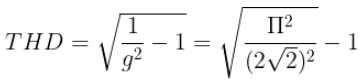In the output voltage the total harmonic distortion THD=48.43%, but as per IEEE, the total harmonic distortion should be 5%.

The fundamental power output of single-phase bridge inverter is

P01= (V01(rms))2/R=I201(rms)R

By using the above formula we can calculate the fundamental power output.

In this way, we can calculate the various parameters of the single-phase half-bridge inverter.

### Single Phase Half Bridge Inverter with R-L Load

The circuit diagram of the R-L load is shown in the below figure.

The circuit diagram of the single-phase half-bridge inverter with R-L load consists of two switches, two diodes, and voltage supply. The R-L load is connected between A point and O point, point A is always considered as positive and point O considered as negative. If the current flow from point A to O then the current will be considered as positive, similarly if the current flow from point to A then the current will be considered as negative.

In the case of R-L Load, the output current will be an exponential function to time and lags the output voltage by an angle.

ϕ= tan-1(ωL/R)

#### Operation of Single Phase Half Bridge Inverter with R- Load

The working operation is based on the following time intervals

(i) Interval I (0<t<t1): In this duration, both the switches are OFF and diode D2 is in reverse bias condition. In this interval, the inductor releases its energy through diode D1, and output current decreases exponentially from its negative max value (-Imax) to zero.

By applying KVL to this time interval will get

The output voltage V0>0; The output current flows in the reverse direction, therefore, i0<0; switch current iS1=0 and diode current iD1= -i0

(ii) Interval II (t1<t<T/2): In this duration, the switch S1 and S2 are closed and S2 is are OFF and both the diodes are in reverse bias condition. In this interval, the inductor starts to store the energy, and output current increases from zero to its positive max value (Imax).

Applying KVL will get

The output voltage V0>0; The output current flows in the forward direction, therefore, i0>0; switch current iS1= i0 and diode current iD1= 0

(iii) Interval III (T/2<t<t2): In this duration, both the switch S1 and S2 are OFF and the diode D is in reverse bias and D2 is in forwarding bias are in reverse bias condition. In this interval, the inductor releases its energy through diode D2. The output current decreases exponentially from its positive max value (Imax) to zero.

Applying KVL will get

The output voltage V0<0; The output current flows in the forward direction, therefore, i0>0; switch current iS1= 0 and diode current iD1= 0

(iv) Interval IV (t2 <t<T): In this duration, the switch S1 is OFF and S2 are closed and the diodes D and D2 are in reverse bias. In this interval, the inductor charged to negative max value (-Imax) to zero.

Applying KVL will get

The output voltage V0<0; The output current flows in the opposite/reverse direction therefore i0<0; switch current iS1= 0 and diode current iD1= 0

Summarization of the time intervals is shown in a below table

 S.NO Time Interval Device Conducts Output Voltage (V0) Output Curent (I0) Switch Current (iS1) Switch Diode (iD1 ) 1 00 I0<0 0 – I0 2 t10 I0>0 I0 0 3 T/20 0 0 4 t2

The output voltage waveform of a single-phase half-bridge inverter with RL load is shown in the below figure.

### Half Bridge Inverter Vs Full Bridge Inverter

The difference between half-bridge inverter and full-bridge inverter is shown in the below table.

 S.NO Half Bridge Inverter Full Bridge Inverter 1 The efficiency is high in half-bridge inverter In full-bridge inverter also, the efficiency is high 2 In half-bridge inverter the output voltage waveforms are square, quasi square or PWM In full-bridge inverter the output voltage waveforms are square, quasi square or PWM 3 The peak voltage in the half-bridge inverter is half of the DC supply voltage The peak voltage in the full-bridge inverter is the same as the DC supply voltage 4 The half-bridge inverter contains two switches The full-bridge inverter contains four switches 5 The output voltage is E0= EDC /2 The output voltage is E0= EDC 6 The fundamental output voltage is E1= 0.45 EDC The fundamental output voltage is E1= 0.9 EDC 7 This type of inverter generates bipolar voltages This type of inverter generates monopolar voltages

The advantages of the single-phase half-bridge inverter are

• Circuit is simple
• Cost is low